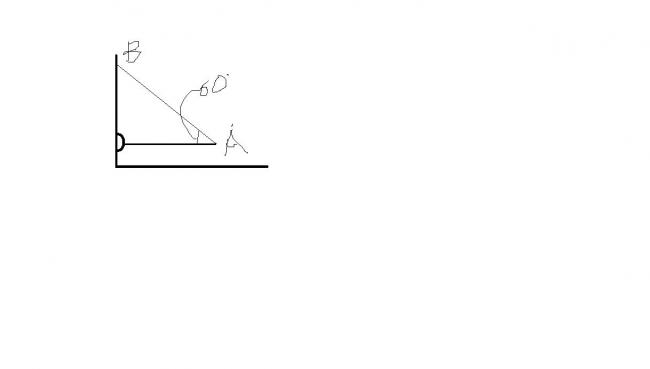# dount no 2

A rod OA of mass 4 kg is held in horizontal position by a massless string AB as shown in the figure,Length of the rod is 2 m .Find :

a.tension in the string

b.net force exerted gy the hingre on the rod•Abhishek Priyam ·

2*Tsin(60)=mg
T=40/√3

for (b)
(conventional way..)
let req force be Fx and Fy

Fx=Tcos(60)=20/√3

Fy+Tsin(60)=mg
Fy=20

F2=400+400/3

F2=1600/3
F=40/√3

•Physics Wallah ·

FROM THE FBD WE CAN GET THAT 2TSIN60=4g=40..SOLVING THIS T=40/âˆš3

•Philip Calvert ·

Tsin60 = mg/2

T √3 = 40

this should give u (a)

b) F = √(Tcos60)2 + (mg/2)2

•peas venkat ·

only T cos 60 will be the force on hinge by the rod

•